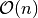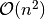# Sorting Analysis¶

Consider a finite collection of orderable elements. Re-arranging that collection, so that the collection is completely ordered is called sorting. There are many techiniques to sort a collection. Following are some of the comparision based Sorting Algorithms.

• Bubble Sort
• Insertion Sort
• Selection Sort
• Merge Sort
• Quick Sort
• Heap Sort

Before looking at the analysis part, we shall examine the Language in built methods to sorting

## sorted(collection,reverse = False[,key])¶

This function takes an iterable as argument, and returns it in sorted form based on key. If key is not given, sorting is done according to default comparision rules. Let’s see the examples and understand the working of sorted(). If reverse is True, reversed collection is returned after sorting.

In :

x = list(range(10))
import random
random.shuffle(x)

In :

x

Out:

[6, 7, 9, 0, 4, 5, 8, 2, 1, 3]

In :

sorted(x)

Out:

[0, 1, 2, 3, 4, 5, 6, 7, 8, 9]

In :

import math
y  = sorted(x,key = lambda x: math.sin(x))  # Sort elements of x in increasing order of their sines
y

Out:

[5, 4, 6, 0, 3, 9, 7, 1, 2, 8]

In :

[math.sin(i) for i in y]

Out:

[-0.9589242746631385,
-0.7568024953079282,
-0.27941549819892586,
0.0,
0.1411200080598672,
0.4121184852417566,
0.6569865987187891,
0.8414709848078965,
0.9092974268256817,
0.9893582466233818]


Note how the elements of sin(y) are in increasing order.

## Standard import statement¶

In :

from openanalysis.sorting import SortingAlgorithm,SortAnalyzer
import numpy as np   # for doing vstack()


SortingAlgorithm is the base class providing the standards to implement sorting algorithms, SortAnalyzer visualizes and analyses the algorithm

## SortingAlgorithm class¶

Any sorting algorithm, which has to be implemented, has to be derived from this class. Now we shall see data members and member functions of this class.

### Data Members¶

• name - Name of the Sorting Algorithm
• count - Holds the number of basic operations performed
• hist_arr - A 2D numpy array, holding the instances of array, as exchange is performed

### Member Functions¶

• __init__(self, name): - Initializes algorithm with a name
• sort(self, array, visualization): - The base sorting function. Sets count to 0. array is 1D numpy array, visualization is a bool indicating whether array has to be vstacked into hist_arr

## An example …. Bubble Sort¶

Now we shall implement the class BubbleSort

In :

class BubbleSort(SortingAlgorithm):                        # Derived from SortingAlgorithm
def __init__(self):
SortingAlgorithm.__init__(self, "Bubble Sort")     # Initializing with name

def sort(self, array, visualization=False):            # MUST have this signature
SortingAlgorithm.sort(self, array, visualization)  # sets self.count to 0
for i in range(0, array.size):                     # Not len(array)
exch = False
for j in range(0, array.size - i - 1):
self.count += 1                            # Increment self.count after each basic operation
if array[j] > array[j + 1]:
array[j], array[j + 1] = array[j + 1], array[j]
exch = True
if visualization:
self.hist_array = np.vstack([self.hist_array, array]) # Save the current state to hist_array
if not exch:
break
if visualization:
self.hist_array = np.vstack([self.hist_array, array])  # Save the final state to hist_array


## SortAnalyzer class¶

This class provides the visualization and analysis methods. Let’s see its methods in detail

• __init__(self, sorter): Initializes visualizer with a Sorting Algorithm.
• sorter is a class, which is derived from SortingAlgorithm
• visualize(self, num=100, save=False): Visualizes the given algorithm with a randomly shuffled array.
• num size of randomly shuffled array
• save is True means animation is saved in output/
• analyze(self, maxpts=1000):
• Plots the running time of sorting algorithm by sorting for 3 cases
• Already Sorted array, reverse sorted array and Shuffled array
• Analysis is done by inputting randomly shuffled integer arrays with size staring from 100, and varying upto maxpts in the steps of 100, and counting the number of basic operations
• maxpts - Upper bound on size of elements chosen for analysing efficiency
In :

bubble_visualizer = SortVisualizer(BubbleSort)

In :

bubble_visualizer.efficiency()As you can see in the above plot, BubbleSort takestime on best case andtime on both avarage and worst cases

You can call the visualize function as shown below and see the ‘mp4’ file saved at output/ folder

bubble_visualizer.visualize(save=True)


## compare(algs)¶

algs is a list of classes derived from SortingAlgorithm. It performs tests and plots the bar graph comparing the number of basic operations performed by each algorithm.

## Why use a class if sorting could be done using a function¶

We have just seen how BubbleSort is implemented. Every sorting algorithm is not as simple as BubbleSort. QuickSort and MergeSort needs several auxiliary methods to work with. If they are scattered throughout the code, they decrease the readability. So it is better to pack everything in a class.

## Example File¶

You can see more examples at Github